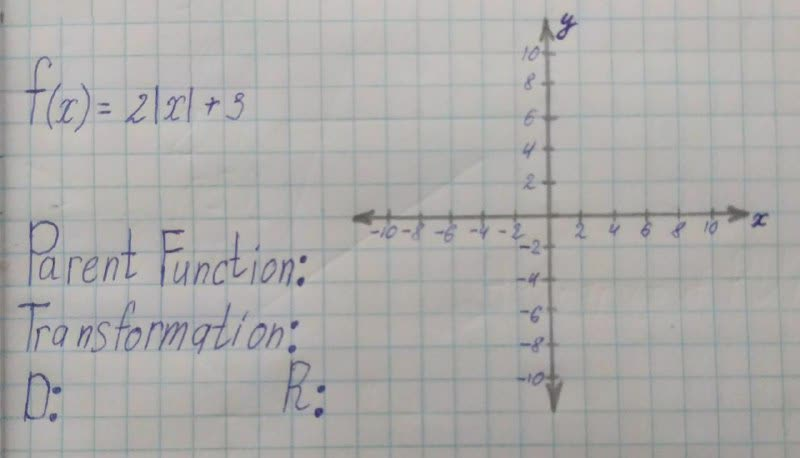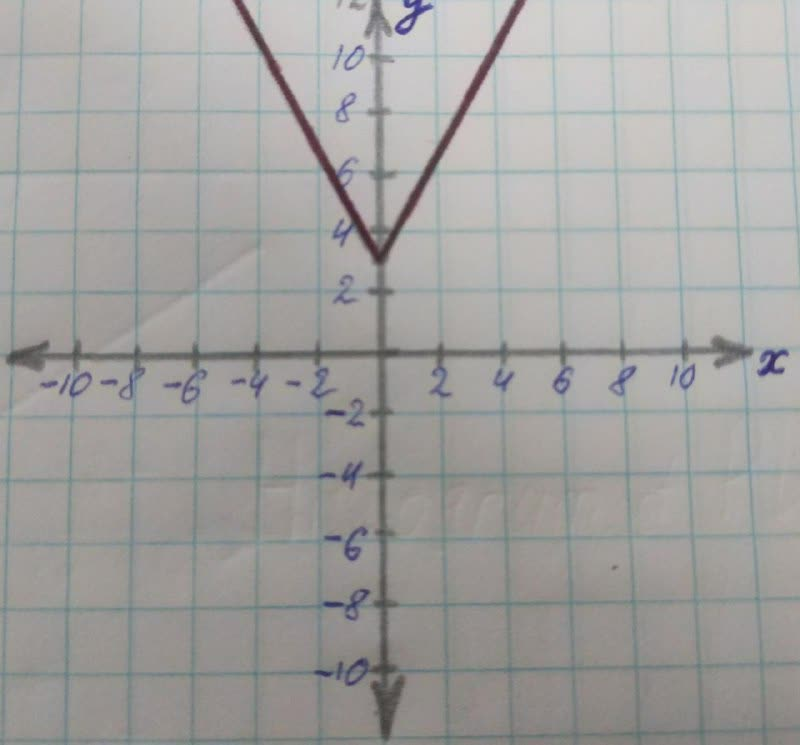# State the parent function, transformations, domain and range. 19610701501.jpgvetrila10 2021-11-22 Answered
State the parent function, transformations, domain and range.• Questions are typically answered in as fast as 30 minutes

### Plainmath recommends

• Get a detailed answer even on the hardest topics.
• Ask an expert for a step-by-step guidance to learn to do it yourself.Robert Harris
Step 1 parent function
Parent function is a function that sustains the basic definition of the whole function. In other words, parent function is the root of the function which isbuild upon it.
the given function is f(x)=2|x|+3
here, the parent function in this equation is f(x)=|x|
Step 2 transformation
first the function |x| is multiplied by
f(x)=2|x|
then 3 is added to the function which lifts the graph along the y-axis by 3 units in the upward direction
f(x)=2|x|+3Step 3 domain
The domain is the set of all values which a function can take so, in this function, all the real value can be taken hence, the domain of this function is R
Step 4 range the range is the set of all the values a function can give when we put the values of x where
$$\displaystyle{x}\in{R}$$ in this equation, the range of a function is all the positive real numbers as mod changes every number into positive number.
so, $$\displaystyle{f{{\left({x}\right)}}}\in{R}^{{+}}$$